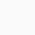# Classical Machine Learning for Financial Engineering

Learn a systemic approach to utilizing classical machine learning models and techniques to gain insights from data sets, and master the tools used in this task.

Classical Machine Learning refers to well established techniques by which one makes inferences from data. This course will introduce a systematic approach (the “Recipe for Machine Learning”) and tools with which to accomplish this task. In addition to the typical models and algorithms taught (e.g., Linear and Logistic Regression) this course emphasizes the whole life cycle of the process, from data set acquisition and cleaning to analysis of errors, all in the service of an iterative process for improving inference.

Our belief is that Machine Learning is an experimental process and thus, most learning will be achieved by “doing”. We will jump-start your experimentation: Engineering first, then math. Early lectures will be a “sprint” to get you programming and experimenting. We will subsequently revisit topics on a greater mathematical basis.

By the end of this course students should be able to:

• apply a systematic approach to solving problems involving analyzing and making inference from data. These problems can come from many different domains but our emphasis will be on Finance.
• make predictions based on financial data
• use alternate data sources such as images and text for prediction
• use these techniques and data for
1. optimizing portfolios
2. risk management
3. streamlining operations

Week 1: Classical Machine Learning: Overview

• What is Machine Learning (ML)?
• ML and Finance; not ML for Finance
• Classical Machine Learning: Introduction
• Supervised Learning
• Our first predictor
• Notational conventions

Week 2: Linear regression. Recipe for Machine Learning

• Linear Regression
• The Recipe for Machine Learning
• The Regression Loss Function
• Bias and Variance

Week 3: Transformations, Classification

• Data Transformations: Introduction and mechanics
• Logistic Regression
• Non-numeric variables: text, images
• Multinomial Classification
• The Classification Loss Function

Week 4: Classification continued, Error Analysis

• Baseline model
• The Dummy Variable Trap
• Transformations
• Loss functions: mathematics

Week 5: More Models: Trees, Forests, Naive Bayes

• Entropy, Cross Entropy, KL Divergence
• Decision Trees
• Naive Bayes
• Ensembles
• Feature Importance

Week 6: Support Vector Machines, Gradient Descent, Interpretation

• Support Vector Classifiers
• Interpretation: Linear Models

Week 7: Unsupervised Learning, Dimensionality Reduction

• Unsupervised Learning
• Dimensionality Reduction
• Clustering
• Principal Components
• Pseudo Matrix Factorization: preview of Deep Learning
0

We will be happy to hear your thoughts